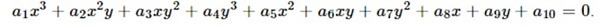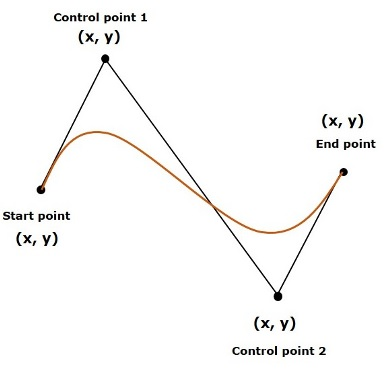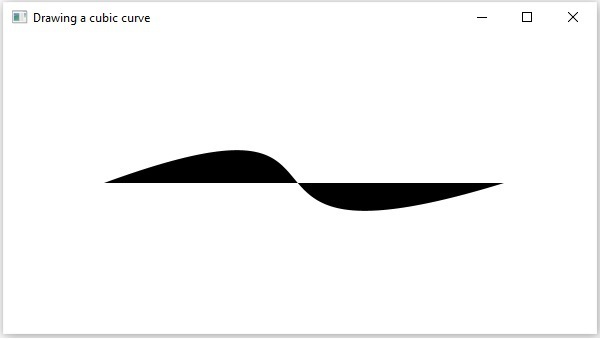# JavaFX - 2D Shapes CubicCurve

A CubicCurve is described by a third-degree polynomial function of two variables, and can be written in the following form −These Bezier curves are generally used in computer graphics. They are parametric curves which appear reasonably smooth at all scales. These curves are drawn based on points on the XY plane.

A cubic curve is a Bezier parametric curve in the XY plane is a curve of degree 3. It is drawn using four points − Start Point, End Point, Control Point and Control Point2 as shown in the following diagram.In JavaFX, a CubicCurve is represented by a class named CubicCurve. This class belongs to the package javafx.scene.shape.

By instantiating this class, you can create a CubicCurve node in JavaFX.

This class has 8 properties of the double datatype namely −

• startX − The x coordinate of the starting point of the curve.

• startY − The y coordinate of the starting point of the curve.

• controlX1 − The x coordinate of the first control point of the curve.

• controlY1 − The y coordinate of the first control point of the curve.

• controlX2 − The x coordinate of the second control point of the curve.

• controlY2 − The y coordinate of the second control point of the curve.

• endX − The x coordinate of the end point of the curve.

• endY − The y coordinate of the end point of the curve.

To draw a cubic curve, you need to pass values to these properties, either by passing them to the constructor of this class, in the same order, at the time of instantiation, as shown below −

```CubicCurve cubiccurve = new CubicCurve(
startX, startY, controlX1, controlY1, controlX2, controlY2, endX, endY);
```

Or, by using their respective setter methods as follows −

```setStartX(value);
setStartY(value);
setControlX1(value);
setControlY1(value);
setControlX2(value);
setControlY2(value);
setEndX(value);
setEndY(value);
```

## Steps to Draw CubicCurve

To draw a Bezier cubic curve in JavaFX, follow the steps given below.

### Step 1: Creating a Class

Create a Java class and inherit the Application class of the package javafx.application and implement the start() method of this class as follows.

```public class ClassName extends Application {
@Override
public void start(Stage primaryStage) throws Exception {
}
}
```

### Step 2: Creating a CubicCurve

You can create a CubicCurve in JavaFX by instantiating the class named CubicCurve which belongs to a package javafx.scene.shape. You can instantiate this class as follows.

```//Creating an object of the class CubicCurve
CubicCurve cubiccurve = new CubicCurve();
```

### Step 3: Setting Properties to the CubicCurve

Specify the x, y coordinates of the four points: start point, end point, control point1 and control point2 of the required curve, using their respective setter methods as shown in the following code block.

```//Setting properties to cubic curve
cubicCurve.setStartX(100.0f);
cubicCurve.setStartY(150.0f);
cubicCurve.setControlX1(400.0f);
cubicCurve.setControlY1(40.0f);
cubicCurve.setControlX2(175.0f);
cubicCurve.setControlY2(250.0f);
cubicCurve.setEndX(500.0f);
cubicCurve.setEndY(150.0f);
```

### Step 4: Creating a Group Object

In the start() method, create a group object by instantiating the class named Group, which belongs to the package javafx.scene.

Pass the CubicCurve (node) object created in the previous step as a parameter to the constructor of the Group class in order to add it to the group as follows −

```Group root = new Group(cubiccurve);
```

### Step 5: Creating a Scene Object

Create a Scene by instantiating the class named Scene which belongs to the package javafx.scene. To this class pass the Group object (root) created in the previous step.

In addition to the root object, you can also pass two double parameters representing height and width of the screen along with the object of the Group class as follows.

```Scene scene = new Scene(group ,600, 300);
```

### Step 6: Setting the Title of the Stage

You can set the title to the stage using the setTitle() method of the Stage class. The primaryStage is a Stage object which is passed to the start method of the scene class as a parameter.

Using the primaryStage object, set the title of the scene as Sample Application as follows.

```primaryStage.setTitle("Sample Application");
```

### Step 7: Adding Scene to the Stage

You can add a Scene object to the stage using the method setScene() of the class named Stage. Add the Scene object prepared in the previous steps using this method as follows.

```primaryStage.setScene(scene)
```

### Step 8: Displaying the Contents of the Stage

Display the contents of the scene using the method named show() of the Stage class as follows.

### Step 9: Launching the Application

Launch the JavaFX application by calling the static method launch() of the Application class from the main method as follows.

```public static void main(String args[]){
launch(args);
}
```

### Example

Following is a program which generates a Bezier CubicCurve using JavaFX. Save this code in a file with the name CubicCurveExample.java.

```import javafx.application.Application;
import javafx.scene.Group;
import javafx.scene.Scene;
import javafx.stage.Stage;
import javafx.scene.shape.CubicCurve;

public class CubicCurveExample extends Application {
@Override
public void start(Stage stage) {
//Drawing a cubic curve
CubicCurve cubicCurve = new CubicCurve();

//Setting properties to cubic curve
cubicCurve.setStartX(100.0f);
cubicCurve.setStartY(150.0f);
cubicCurve.setControlX1(400.0f);
cubicCurve.setControlY1(40.0f);
cubicCurve.setControlX2(175.0f);
cubicCurve.setControlY2(250.0f);
cubicCurve.setEndX(500.0f);
cubicCurve.setEndY(150.0f);

//Creating a Group object
Group root = new Group(cubicCurve);

//Creating a scene object
Scene scene = new Scene(root, 600, 300);

//Setting title to the Stage
stage.setTitle("Drawing a cubic curve");

stage.setScene(scene);

//Displaying the contents of the stage
stage.show();
}
public static void main(String args[]){
launch(args);
}
}
```

Compile and execute the saved java file from the command prompt using the following commands.

```javac CubicCurveExample.java
java CubicCurveExample
```

On executing, the above program generates a JavaFX window displaying a Bezier cubic curve as shown below.javafx_2d_shapes.htm